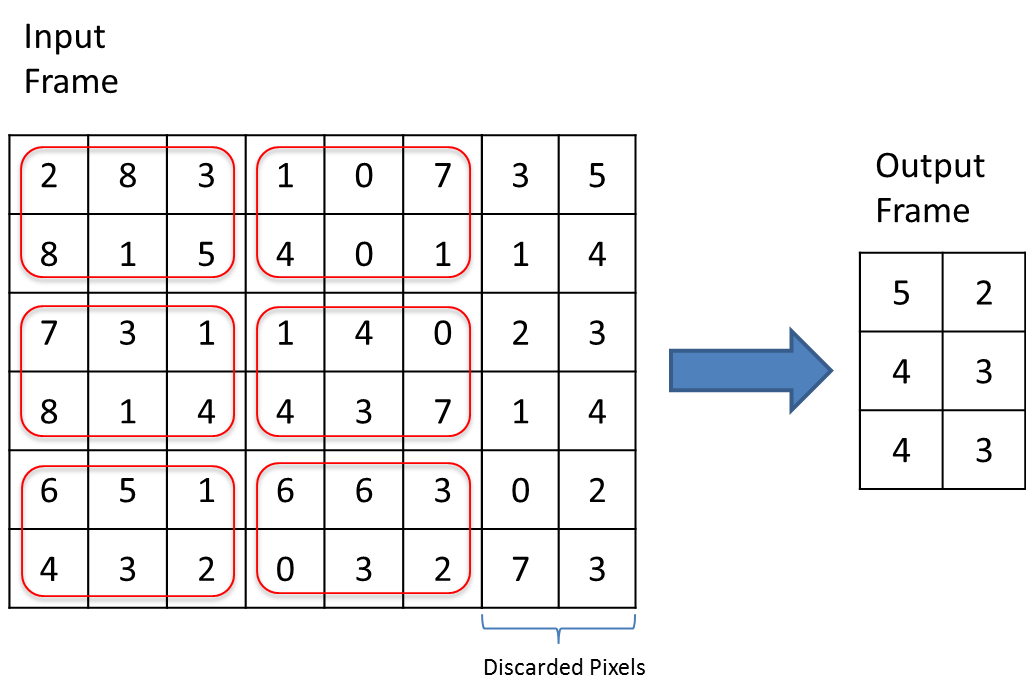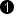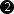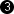## Operator SampleDn

The operator SampleDn reduces the image size by downsampling the input image. The image size downsampling factor can be individually defined for the width and height using any positive integer number up to 128. This downsmpling factor is defined using parameters XSampleDown and YSampleDown. The output pixels are computed by averaging the joint pixels.

The operator functions properly only on rectangular images, i.e. images whose lines are of equal length. Images with variable line length within the same image will lead to wrong results.

If the down sampling parameters are set to a value that exceeds the width/height of the input image, the resulting image will be an empty image.

If the actual image width/height is not divisible by the correspondent downsampling parameter value, the operator will discard the remaining pixels. See the following figure for explanation.If the width of an incoming image divided by XSampleDown is not a multiple of the parallelism and is smaller than the calculated max. image width of the output then the image lines are expanded by appending dummy pixels so the resulting line length is a multiple of the parallelism. The value of the inserted dummy pixels is undefined.

Operator Restrictions

• The lines of each input image must have the same length. Thus images with varying line lengths are not allowed.

• For color pixels, each color component is processed individually.

• If the actual image width is less than XSampleDown, an empty image is output.

• If the actual image height is less than YSampleDown, an empty image is output.

### I/O Properties

Property Value
Operator Type P
Input Link I, data input
Output Link O, data output

### Supported Link Format

Bit Width [1, 64]as I
Arithmetic {unsigned, signed} as I
Parallelism any as I
Kernel Columns any as I
Kernel Rows any as I
Img Protocol {VALT_IMAGE2D, VALT_LINE1D} as I
Color Format any as I
Color Flavor any as I
Max. Img Width any autoMax. Img Height any autoThe range of the input bit width is [1, 64] for unsigned values. For signed inputs, the range is [2, 64]. For unsigned color inputs [3, 63] and for signed color inputs [6, 63]. The maximum image dimension at the output is automatically determined according to the following formulas:

### Parameters

XSampleDown
Type static parameter
Default
Range [1, 128]

This parameter defines the down sampling factor for the image width.

The downsampling is limited to

YSampleDown
Type static parameter
Default
Range [1, 128]

This parameter defines the down sampling factor for the image height.

The downsampling is limited to

### Examples of Use

The use of operator SampleDn is shown in the following examples:

• 'Downsampling'

Examples - The input image is downsampled i.e. reduced in size by 4x4.

• 'Downsampling 3x3'

Examples - Downsampling by factor 3x3 without the use of operator SampleDn.